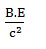# Mp denotes the mass of a proton and Mn that of a neutron. A given nucleus, of binding energy B, contains Z protons and N neutrons. The mass M(N, Z) of the nucleus is given by (c is the velocity of light) (a)    M(N, Z) = NMn + ZMp + B/c2(b)    M(N, Z) = NMn + ZMp - Bc2 (c)    M(N, Z) = NMn + ZMp + Bc2 (d)    M(N, Z) = NMn + ZMp – B/c2Aditi Chauhan
8 years ago

(d)

Mass defect =Mass of nucleus = Mass of proton + mass of neutron - mass defect